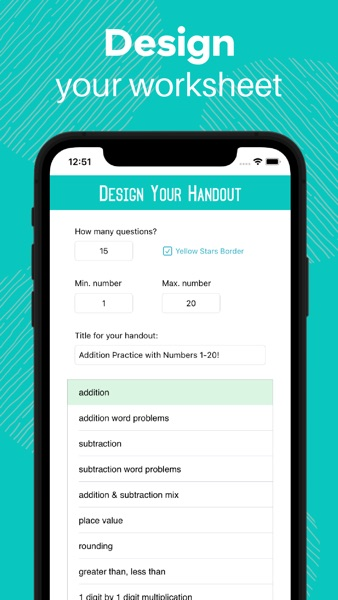Updated:
Version:
1.0
Size:
5.1 MB
Category:
Education
Required:
iOS
Rating:
Publish:
2023-03-14
Price:
\$2.99
Developer:

### Math Worksheet Maker App DESCRIPTION

Math Worksheet Maker is an easy-to-use math worksheet generator perfect for teachers, tutors, and parents! With our customization features, you won’t get the same worksheet twice. Every worksheet is unique.

In less than a minute you can create a beautiful handout, print it, share it, save it, and have it ready to use!

FEATURES

1. Customizable

* Enter the number of questions you would like for your handout.
* Enter the minimum number and the maximum number that can appear in your math problems. For example, if you would like to create a handout for subtraction using numbers 1-100, enter “1” as your minimum number and “100” as your maximum number.
* You can use negative numbers too!
* Create a title for your worksheet (optional). E.g., “Adding Fractions: Quiz 2” or “Ellie’s Multiplication Practice.”
* Title space can also be used for directions.
* Choose a fun border from over 50 different options! (optional)
* Include an answer key (optional).

2. Over 30 Different Math Functions

* subtraction
* subtraction word problems
* place value
* rounding
* greater than / less than (number comparisons)
* 1 digit by 1 digit multiplication
* 2 digits by 1 digit multiplication
* 3 digits by 1 digit multiplication
* 2 digits by 2 digits multiplication
* 3 digits by 2 digits multiplication
* 3 digits by 3 digits multiplication
* times tables
* multiplication (fully randomized)
* division by 1 digit
* division by 2 digits
* subtraction with decimals
* addition and subtraction with decimals mix
* multiplication with decimals
* improper fractions
* subtraction with fractions
* multiplication with fractions
* division with fractions
* comparing fractions (to other fractions or to decimals)
* order of operations practice
* one variable algebra (easy)
* one variable algebra (regular)

(Note: One variable algebra does not include graphing questions. One variable algebra (regular) focuses on solving for x, especially using the quadratic equation.)

3. Share, Save, or Print!
Once you’ve created your worksheet you can save it, share it via text or email, or send it right to the printer!

All worksheets are copyright of LearnEd Apps LLC and are not to be used for resale or redistribution online or in print.